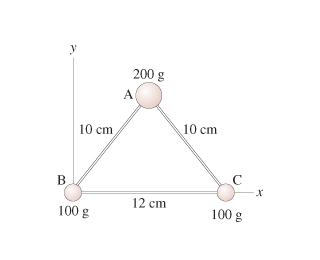# Problem: Find the y-coordinate.Express your answer to two significant figures and include the appropriate units.

###### FREE Expert Solution

Center of mass about an axis is given by:

$\overline{)\begin{array}{rcl}\mathbf{\sum }_{\mathbf{n}\mathbf{=}\mathbf{1}}^{\mathbf{n}\mathbf{=}\mathbf{i}}{\mathbf{m}}_{\mathbf{i}}{\mathbf{y}}_{\mathbf{i}}& {\mathbf{=}}& \mathbf{\left(}\mathbf{\sum }_{\mathbf{n}\mathbf{=}\mathbf{1}}^{\mathbf{n}\mathbf{=}\mathbf{i}}{\mathbf{m}}_{\mathbf{i}}\mathbf{\right)}\overline{\mathbf{y}}\end{array}}$

The two 100 g masses are located at y = 0 cm. The 200 g mass is located at the height of the triangle. Using Pythagoras theorem, the height of the triangle is:

h = [hyp2 - (base/2)2](1/2) = (102 - 62)(1/2) = 8 cm###### Problem DetailsFind the y-coordinate.

Express your answer to two significant figures and include the appropriate units.